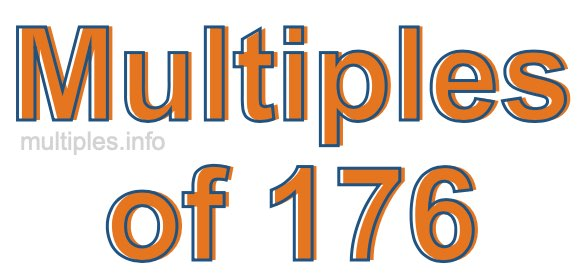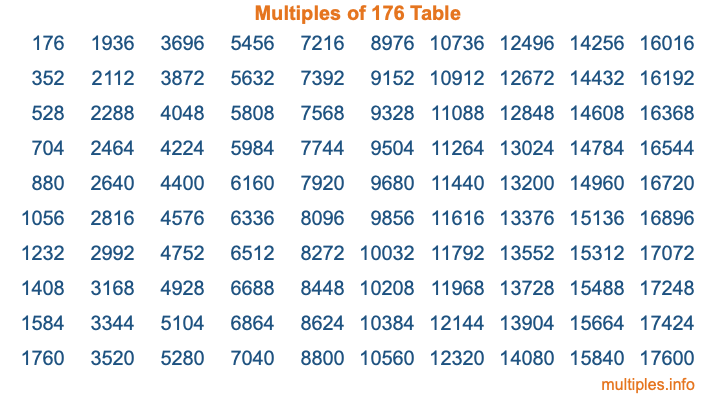Multiples of 176Welcome to the Multiples of 176 page. Here we will first teach you everything you will ever need to know about the multiples of 176, and then give you a study guide summary of everything we taught you to make sure you remember it all. Use this page to look up facts and learn information about the multiples of 176. This page will make you a multiples of one hundred seventy-six expert!

Definition of Multiples of 176
Multiples of 176 are all the numbers that when divided by 176 equal an integer. Each of the multiples of 176 are called a multiple. A multiple of 176 is created by multiplying 176 by an integer.

Therefore, to create a list of multiples of 176, you start with 1 multiplied by 176, then 2 multiplied by 176, then 3 multiplied by 176, and so on for as long as you want. Thus, the list of the first five multiples of 176 is 176, 352, 528, 704, and 880. To see a larger list of multiples of 176, see the printable image of Multiples of 176 further down on this page. We also have a category where you can choose any nth multiple of 176.

Multiples of 176 Checker
The Multiples of 176 Checker below checks to see if any number of your choice is a multiple of 176. In other words, it checks to see if there is any number (integer) that when multiplied by 176 will equal your number. To do that, we divide your number by 176. If the the quotient is an integer, then your number is a multiple of 176.

Is  a multiple of 176?

Least Common Multiple of 176 and ...
A Least Common Multiple (LCM) is the lowest multiple that two or more numbers have in common. This is also called the smallest common multiple or lowest common multiple and is useful to know when you are adding our subtracting fractions. Enter one or more numbers below (176 is already entered) to find the LCM.

Check out our LCM Calculator if you need more details about the Least Common Multiple or if you need the LCM for different numbers for adding and subtraction fractions.

nth Multiple of 176
As we stated above, 176 is the first multiple of 176, 352 is the second multiple of 176, 528 is the third multiple of 176, and so on. Enter a number below to find the nth multiple of 176.

th multiple of 176

Multiples of 176 vs Factors of 176
176 is a multiple of 176 and a factor of 176, but that is where the similarities end. All postive multiples of 176 are 176 or greater than 176. All positive factors of 176 are 176 or less than 176.

Below is the beginning list of multiples of 176 and the factors of 176 so you can compare:

Multiples of 176: 176, 352, 528, 704, 880, etc.

Factors of 176: 1, 2, 4, 8, 11, 16, 22, 44, 88, 176

As you can see, the multiples of 176 are all the numbers that you can divide by 176 to get a whole number. The factors of 176, on the other hand, are all the whole numbers that you can multiply by another whole number to get 176.

It's also interesting to note that if a number (x) is a factor of 176, then 176 will also be a multiple of that number (x).

Multiples of 176 vs Divisors of 176
The divisors of 176 are all the integers that 176 can be divided by evenly. Below is a list of the divisors of 176.

Divisors of 176: 1, 2, 4, 8, 11, 16, 22, 44, 88, 176

The interesting thing to note here is that if you take any multiple of 176 and divide it by a divisor of 176, you will see that the quotient is an integer.

Multiples of 176 Table
Below is an image of the first 100 multiples of 176 in a table. The table is in chronological order, column by column. The first column has the first ten multiples of 176, the second column has the next ten multiples of 176, and so on.The Multiples of 176 Table is also referred to as the 176 Times Table or Times Table of 176. You are welcome to print out our table for your studies.

Negative Multiples of 176
Although not often discussed or needed in math, it is worth mentioning that you can make a list of negative multiples of 176 by multiplying 176 by -1, then by -2, then by -3, and so on, to get the following list of negative multiples of 176:

-176, -352, -528, -704, -880, etc.

Multiples of 176 Summary
Below is a summary of important Multiples of 176 facts that we have discussed on this page. To retain the knowledge on this page, we recommend that you read through the summary and explain to yourself or a study partner why they hold true.

There are an infinite number of multiples of 176.

A multiple of 176 divided by 176 will equal a whole number.

176 divided by a factor of 176 equals a divisor of 176.

The nth multiple of 176 is n times 176.

The largest factor of 176 is equal to the first positive multiple of 176.

176 is a multiple of every factor of 176.

176 is a multiple of 176.

A multiple of 176 divided by a divisor of 176 equals an integer.

176 divided by a divisor of 176 equals a factor of 176.

Any integer times 176 will equal a multiple of 176.

Multiples of a Number
Here you can get the multiples of another number, all with the same attention to detail as we did for multiples of 176 on this page.

Multiples of
Multiples of 177
Did you find our page about multiples of one hundred seventy-six educational? Do you want more knowledge? Check out the multiples of the next number on our list!